# Introduction to Hyperbolas

Go back to  'Hyperbola'

As already mentioned earlier, a hyperbola is the locus of a moving point such that the ratio of its distance from a fixed point to its distance from a fixed line is constant, i.e, it is a conic section with eccentricity  $$e > 1.$$

In this section, we will follow a first principles approach to derive the basic equation representing a hyperbola (the approach followed is analogous to the one we used to derive the equation of the ellipse).

Assume F to be the fixed point (the focus) and L to be the fixed line (the directrix) of the hyperbola whose equation we wish to determine. For any point P lying on the hyperbola.

$\frac{{({\text{Distance of }}P{\text{ from }}F{\rm{)}}}}{{{\text{(Distance of }}P{\text{ from the line}}\;\;L{\rm{)}}}} = e$

must always be satisfied: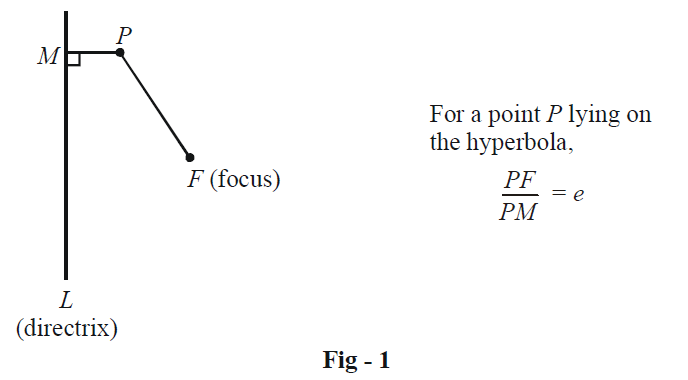As in the case of deriving the equation of the ellipse, we draw here a line L' through F perpendicular to L meeting L in N. On this line, we can find two points A and A' which divide the line segment FN internally and externally (respectively) in the ratio e: 1, as shown in the figure below: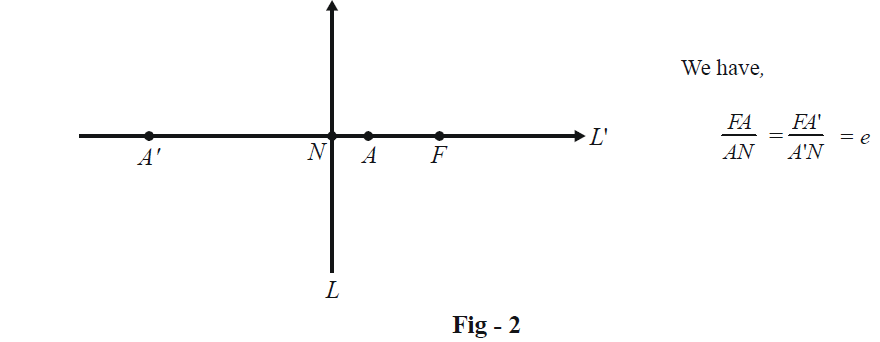Thus, A and A' must lie on the hyperbola by definition.

As in the case of deriving the ellipse’s equation, we take the coordinate axes here to be parallel to L and L', and the origin O to be at the mid-point of A and A'. A and A' can be assumed to have the coordinates (a, 0) and (–a, 0) so that AA' = 2a.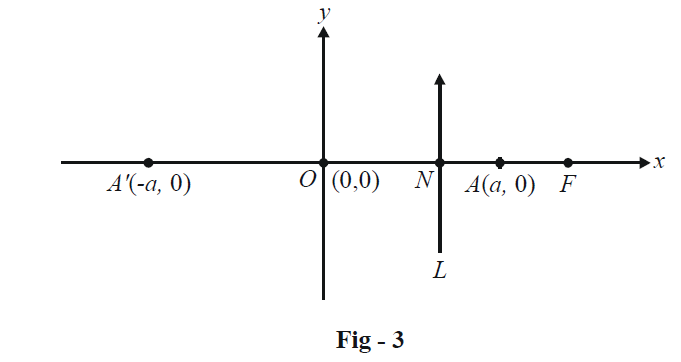The coordinates of N and F can be found as follows. Let e be the eccentricity of the hyperbola. We have,

\begin{align}&AA' = 2a = A'F - AF = eA'N - eAN \ \\\\&\qquad\qquad\qquad\qquad\quad\quad= e(A'N - AN) \\\\&\qquad\qquad\qquad\qquad\quad\quad= e(A'O + ON - AN) \\\\&\qquad\qquad\qquad\qquad\quad\quad= e(AO + ON - AN)\quad(\because \,\,A'O = AO = a) , \\\\&\qquad\qquad\qquad\qquad\quad\quad= 2e(ON) \\\\\end{align}

This gives

$ON = \frac{a}{e}$

Also,

\begin{align}&\;\;\;\;\,\,\,\,\,\,\,\,\,\,\,\,\,\,AF\,\, = e(AN)\\\\\,\,\,\,\,\,\,\,\,\,\,\,\,\,\,\,\,\,\,\,\,\,\,\, &\qquad\qquad\;\;\;\;= e\left( {a - \frac{a}{e}} \right)\\\\\,\,\,\,\,\,\,\,\,\,\,\,\,\,\,\,\,\,\,\,\,\,\,\, &\qquad\qquad\;\;\;\;= ae - a\\\\ &\Rightarrow \,\,\,\,\,\,\,\,\,\,OF\,\, = \,AF + a\\\\,\,\,\,\,\,\,\,\,\,\,\,\,\,\,\,\,\,\,\,\,\,\,\, &\qquad\qquad\;\;\;\;= ae\end{align}

Thus, the coordinates of N and F are \begin{align}\left( {\frac{a}{e},\,\,0} \right)\end{align} and $$\left( {ae,\,\,0} \right).$$

Again, as in the case of the ellipse, it looks as if a hyperbola should posess two foci and two directrices. This will become evident once we write the equation of the hyperbola.

Let $$P(x,\,y)$$ be any point on the hyperbola. By definition,

$\frac{{PF}}{{({\text {Distance of }}\;P\;{\text{ from }}L{\rm{)}}}} = e$

\begin{align}&\Rightarrow \quad{(x - ae)^2} + {y^2} = {e^2}{\left( {x - \frac{a}{e}} \right)^2} \\\\&\Rightarrow \quad{x^2}(1 - {e^2}) + {y^2} = {a^2}(1 - {e^2}) \\\\&\Rightarrow\quad \frac{{{x^2}}}{{{a^2}}} - \frac{{{y^2}}}{{{a^2}({e^2} - 1)}} = 1 \\\\&\Rightarrow \quad\boxed{\frac{{{x^2}}}{{{a^2}}} - \frac{{{y^2}}}{{{b^2}}} = 1}\qquad \quad \text{where }\;\; {b^2} = {a^2}({e^2} - 1) \end{align}

The equation obtained for the hyperbola makes it quite evident that another focus F ' and another directrix L' will exist symmetrically to the left of the origin. The following diagram shows clearly the plot of the hyperbola given by the equation above. Observe it carefully.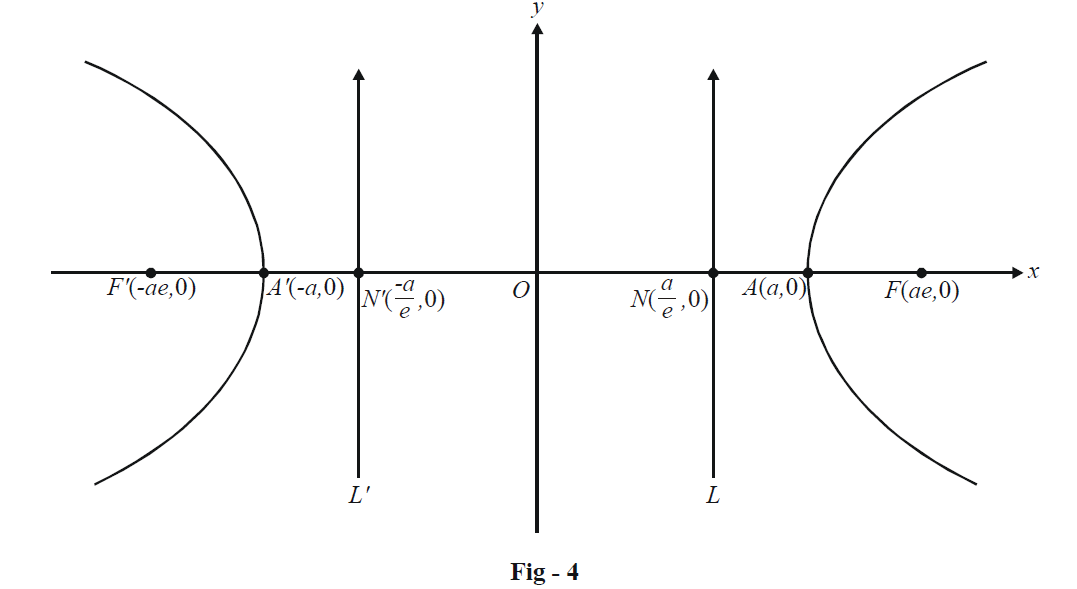Thus, we see that a hyperbola has two disjoint curves. This should hardly be surprising once you recall how the hyperbola is obtained by cutting a double-cone (refer to the Introduction to Parabolas). You are urged to verify the validity of the curve we have plotted above. For any point P on either segment of the hyperbola, check explicitly that the following relations always hold:

$\frac{{PF}}{{{\rm{Distance\; of\; }}P{\rm{ \;from \;}}L}} = \frac{{PF'}}{{{\rm{\;Distance\; of\; }}P{\rm{\; from \;}}L'}} = e$

Here is a brief summary on the terminology we use for a hyperbola (we are using Fig - 4 as a reference)

 Centre : The point O Vertices : The points A (a, 0) and A'(–a, 0) Transverse axis : The segment joining the vertices; AA' above. Its length is 2a. Conjugate axis : The line through the center perpendicular to the transverse axis (the y-axis above). Although the hyperbola never intersects the conjugate axis, we still say that the ‘length’ of the conjugate axis is 2b. You should not get worried about this; it is said just to maintain uniformity.

Now we come to a very significant and interesting property of any hyperbola.

Consider $$P(x,\,y)$$ as any point on the hyperbola. Its focal distances are PF and PF', as shown below: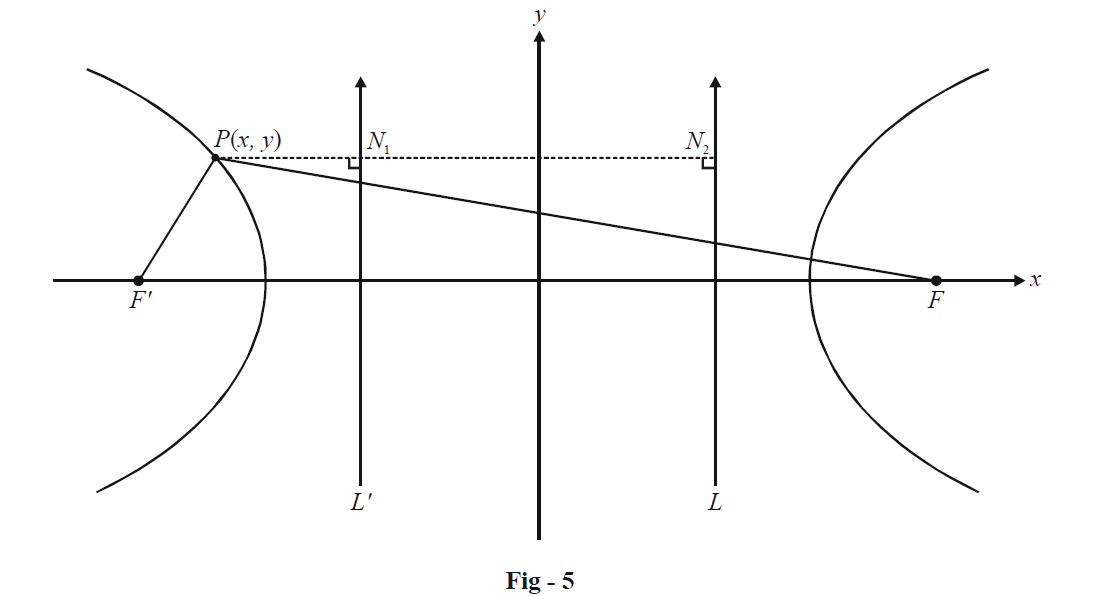Referring to the figure above, observe that

\begin{align}&PF = e(P{N_2}) = e\left( {\frac{a}{e} - x} \right) \\\,\,\,\,\,\,\,\,\,\,\,\,\,\,\,\,\,\,\,\,\,\,\,\,\,\,\,\,\, &\qquad\qquad\qquad= a - ex \\&PF' = e(P{N_1}) = e\left( {\frac{{ - a}}{e} - x} \right) \\\,\,\,\,\,\,\,\,\,\,\,\,\,\,\,\,\,\,\,\,\,\,\,\,\,\,\,\,\,\, &\qquad\qquad\qquad\;= - a - ex \\&\Rightarrow\ \boxed{\left| {PF - PF'} \right| = 2a = ({\text{The transverse axis)}}} \\\end{align}

This equality states that for any point P on the hyperbola, the difference between its focal distances is always a constant, equal to the length of the transverse axis. Thus, a hyperbola can alternatively be defined as follows:

$\boxed{\text {A hyperbola is the locus of a moving point such that the} \;\mathbf{difference}\; \text{of its distances from}\\\text {two fixed points is always constant.The two fixed points are called the foci of the}\\\text {hyperbola.}}$

Contrast this with the definition of the ellipse where we had the sum of focal distances (instead of difference) as constant.

As in the case of the ellipse, we have:

\begin{align}& \mathbf{Focal}\text{ }\mathbf{distances}\ \mathbf{of}~\mathbf{P}(x,y)~~~~~:~~\,\,\,{{d}_{1}}=e(PF)=e\left( x-\frac{a}{e} \right)=ex-a~~\\ &\qquad\qquad\qquad\qquad\qquad\qquad\qquad\quad{{d}_{2}}=e(P{F}')=e\left( x+\frac{a}{e} \right)=ex+a \end{align}

Latus-Rectum : The chord(s) of the hyperbola passing through the focus F(or F ') and perpendicular to the transverse axis. The length of the latus-rectum can be evaluated by substituting $$x = \pm ae$$ in the equation for the hyperbola:

\begin{align}&\qquad \frac{{{x^2}}}{{{a^2}}} - \frac{{{y^2}}}{{{a^2}({e^2} - 1)}} = 1\\ &\Rightarrow\quad {e^2} - \frac{{{y^2}}}{{{a^2}({e^2} - 1)}} = 1\\ &\Rightarrow\quad {y^2} = {a^2}{({e^2} - 1)^2} = \frac{{{a^2} \cdot {b^4}}}{{{a^4}}} = \frac{{{b^4}}}{{{a^2}}}\\ &\Rightarrow \quad y = \pm \frac{{{b^2}}}{a}\end{align}

Thus, the length of the latus-rectum is \begin{align}\frac{{2{b^2}}}{a}.\end{align}

We discussed in the unit on Ellipse that an ellipse with center at $$(\alpha ,\,\,\beta )$$ instead of the origin and the major and minor axis parallel to the coordinate axes will have the equation

\begin{align}&\,\,\,\,\,\,\,\,\,\,\,\,\,\,\,\,\,\,\,\,\,\,\,\,\,\,\,\,\,\,\,\frac{{{{(x - \alpha )}^2}}}{{{a^2}}} + \frac{{{{(y - \beta )}^2}}}{{{b^2}}} = 1\\\\&\quad or\,\,\,\,\,\,\,\,\,\,\,\,\,\,\,\,\,\,\,\,\,\,\,\,\,\,\frac{{{X^2}}}{{{a^2}}} + \frac{{{Y^2}}}{{{b^2}}} = 1\end{align}

where $$X \to x - \alpha \;\;\text{and}\;\;Y \to y - \beta .$$

The same holds true for a hyperbola. Any hyperbola with center at $$(\alpha ,\,\,\beta )$$ and the transverse and conjugate axis parallel to the coordinate axes will have the form

\begin{align}&\,\,\,\,\,\,\,\,\,\,\,\,\,\,\,\,\,\,\,\frac{{{{(x - \alpha )}^2}}}{{{a^2}}} - \frac{{{{(y - \beta )}^2}}}{{{b^2}}} = 1\\\\&or\,\,\,\,\,\,\,\,\,\,\,\,\,\,\,\frac{{{X^2}}}{{{a^2}}} - \frac{{{Y^2}}}{{{b^2}}} = 1\end{align}

where $$X \to x - \alpha \;\;\text{and}\;\;Y \to y - \beta .$$

We can, using the definition of a hyperbola, write the equation of any hyperbola with an arbitrary focus and directrix, but we’ll rarely have the occasion to use it.

Hyperbolas
grade 11 | Questions Set 1
Hyperbolas
Hyperbolas
grade 11 | Questions Set 2
Hyperbolas